# Tikhonov cube

(diff) ← Older revision | Latest revision (diff) | Newer revision → (diff)
The topological product ofcopies of the unit intervalof the real line, whereis an arbitrary cardinal number. It is denoted by. The Tikhonov cube was introduced by A.N. Tikhonov in 1929. Ifis a natural number, then the Tikhonov cubeis the unit cube in-dimensional Euclidean space, and its topology is induced from the scalar-product metric. If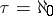is the cardinality of the natural numbers, then the cube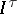is homeomorphic to the Hilbert cube. For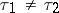, the Tikhonov cubes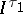and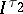are not homeomorphic: Ifis an infinite cardinal number, thenis the weight of(cf. Weight of a topological space), while ifis a natural number, thenis the dimension of. Two properties of the Tikhonov cubesare particularly important: the compactness of each of them, independently of, and their universality with respect to completely-regular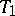-spaces of weight not exceeding: Each such space is homeomorphic to some subspace of. Compact Hausdorff spaces of weight not exceedingare homeomorphic to closed subspaces of the Tikhonov cube. Thus, these two operations — taking topological products and transition to closed subspaces — suffice to obtain every compact space from a single, particularly simple, standard space — the interval. A remarkable consequence of the compactness of Tikhonov cubes is the compactness of the unit ball in a Banach space equipped with the weak topology. The universality of the Tikhonov cubes, and the simplicity of their definition, makes them important standard objects in general topology. However, the topological structure of the Tikhonov cubes is far from trivial. In particular, the cube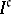, whereis the cardinality of the continuum, is separable, although it containspoints; its weight is. A surprising fact is that the Suslin number of each Tikhonov cubeis countable, independently of, i.e. every collection of pairwise-disjoint open sets inis countable. Although a Tikhonov cube contains many convergent sequences, these do not suffice to directly describe the closure operator in a Tikhonov cube.